# 2 Ways to Subtract Or Add Months, Years And Days To Date In Excel

July 22, 2022
1.8K Views
0

A free Office suite fully compatible with Microsoft Office

A free Office suite fully compatible with Microsoft Office

Imagine you want to add five months to a project's schedule date to see what the final closing date would be or you want to figure out how long a certain activity in a list of project activities will take to complete so you need to add months to date in excel. Excel worksheet functions that are made expressly to operate with dates can be used to subtract or add months to date in excel. Also you can add years and days to a date as well.

Here are the tutorials for how you can subtract or add months, years and days to date.

The function, called EDATE function is used to subtract or add months to date in excel. EDATE function is particularly helpful in financial modelling and altering timelines.

The EDATE function need two inputs, the start date and the number of months you want to subtract or add to the date.

Here is the stepwise tutorial on how you can use the EDATE function to subtract or add months to date in excel.

1. Enter the dates you want to alter in a column. Also, make sure that dates you are entering are formatted properly.2. Enter the number of months you have to add or subtract in adjacent column.

3.To add months to date in excel use a positive number and in order to subtract months use negative number.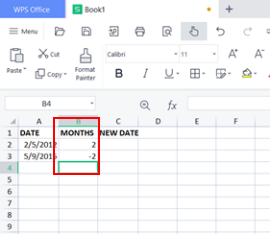1. Format the output cell as date. Select the cell where you want to display your new date.

2. Use the formula: =EDATE(cell having start date, cell having number of months).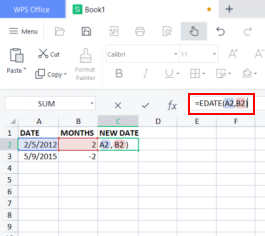1. Press the return key. This should add months to date in excel successfully.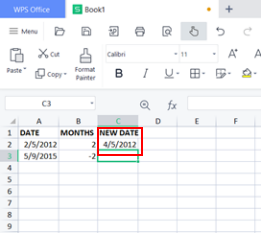1. Follow the same procedure with other dates or use auto fill option.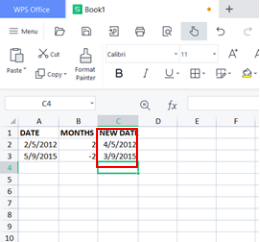Now you can subtract or add months to date in excel.

# Add or subtract years from date

Here is the stepwise tutorial on how you can add or subtract years from date.

1. Enter the dates you want to alter in a column.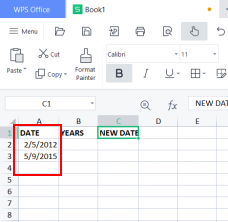1. Enter the number of years you have to add or subtract in adjacent column.

2. To add years to date in excel use a positive number and in order to subtract years use negative number.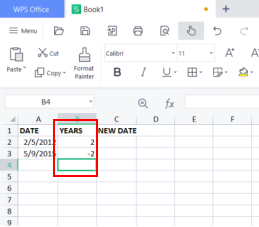1. Select the cell where you want to display your new date.

2. Use the formula: =DATE(YEAR(A2)+B2,MONTH(A2),DAY(A2). Where A2 is the cell having original date and B2 is the cell having number of years to add or subtract.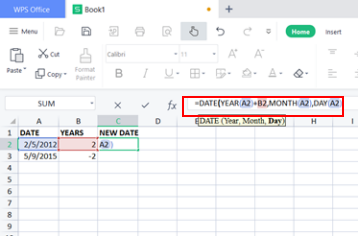1. Press the return key.This should display the new date.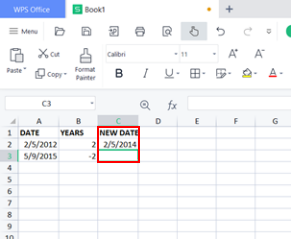1. Follow the same procedure with other dates or use auto fill option.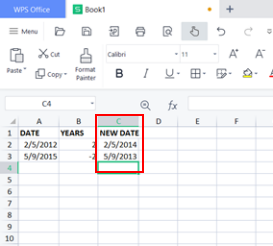Now you can subtract or add years from date n excel.

# Subtract Or Add Days From Date

Here is the stepwise tutorial on how you can add or subtract days from date.

1. Enter the dates you want to alter in a column.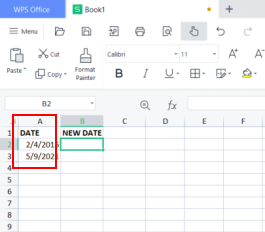1. To add days to date in excel use a positive number and in order to subtract days use negative number.

2. Select the cell where you want to display your new date.

3. Use the formula: =cell having original date+days to add or =cell having originaldate-days to subtract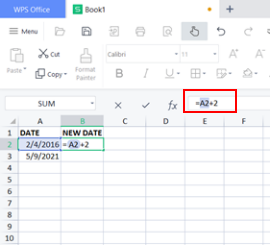1. Press the return key. This should display the new date.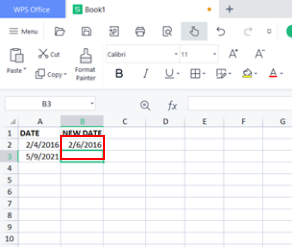Now you can subtract or add days from date in excel.

The above tutorials are applicable on all versions of WPS including 2016/2019 versions. All the versions are available on multiple platforms such as window and mac. Also, in order to use excel you don’t need to be online as it can be used without internet as well.